# Module PorousMedia

## Overview

Module Porous Media is responsible for handling all water fluxes in soil, including water transport due to the balance between pressure (gravity and suction) and resistance trough the medium, infiltration, evapotranspiration and link with the river (groundwater flow). Soil fluxes are calculated by the Buckingham-Darcy equation (Jury et al,1991) and the connections with surface runoff and river are done using the same formulation where the surface water Head is the water level.

## Main Processes

### Porous Media Geometry

Porous Media is a 3D domain delimited in its upper limit by topography and lower limit by soil bottom (defined by user). In terms of soil definition it can be defined vertical horizons to correspond to real soil horizons with different hydraulic carachteristics. See the picture below for information.

### Water Flow

Soil contains a large distribution of pore sizes and channels through which water may flow. In general, the water flow determination is based on the mass conservation and momentum equation Equations. In the case of soil it is assumed that acceleration is close to zero since velocities are very low; therefore the balance is reduced to the forces of pressure, gravity and viscous. The equation that describes the flow through soil is the Buckingham Darcy equation (Jury et al,1991).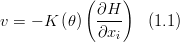$v=-K\left ( \theta \right )\left ( \frac{\partial H}{\partial x_i} \right )\,\,\,\,(1.1)$

where:

 v is the water velocity at the cell interface (m/s) H is the hydraulic head (m) θ is the water content (m3/m3) K is the hydraulic conductivity (m/s) x_i is direction i

The hydraulic head is given by the formula: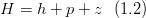$H=h+p+z\,\,\,\,(1.2)$

where:

 h is the hydraulic head (m) p is hydrostatic pressure (m) z is the topography (m)

When in saturated conditions, hydraulic head is zero and hydrostatic pressure may occur (if water is at rest or decelerating). In unsaturated conditions, hydrostatic pressure is zero and hydraulic head exists.

The soil is a very complex system, made up of a heterogeneous mixture of solid, liquid, and gaseous material. The liquid phase consists of soil water, which fills part or all of the open spaces between the soil particles. Therefore it is possible to divide the soil into two parts:

• Saturated soil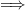$\Longrightarrow$ The soil pores are filled with water
• Unsaturated one$\Longrightarrow$ The soil pores are filled with water and air

In the first case, the equation of Buckingham Darcy is simplified to the Darcy law and the parameter associated with its resolution are connected with the saturated layer. On the other hand for the resolution of the equation (1.1) a description of the characteristics of the unsaturated layer is needed.

Many vadose flow and transport studies require description of unsaturated soil hydraulic proprieties over a wide range of pressure heads. The hydraulic proprieties are described using the porous size distribution model of Maulem (1976) for hydraulic conductivity in combination with a water retention function introduced by Van Genuchten (1980).

#### Water content

Water content is the quantity of water contained in the soil (called soil moisture). It is given as a volumetric basis and it is defined mathematically as: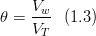$\theta = \frac{V_w}{V_T}\,\,\,\,(1.3)$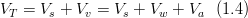$V_T = V_s + V_v = V_s + V_w + V_a\,\,\,\,(1.4)$

where: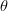$\theta$ is water content (m 3/m 3)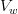$V_w$ is the volume of water (m s3)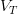$V_T$ is the total volume (m 3)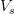$V_s$ is the soil volume (m 3)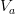$V_a$ is the air space (m 3)

### Initial Condition

The user may define a water content initialization or choose the model to compute in the unsaturated area heads so that soil water is at Field Capacity. The below explains the latter.

Once determined the aquifer level (water table) the water content is associated at each cells by the following criteria:

• If the cell is located above the water table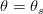$\theta=\theta_{s}$
• If the cell is located over the water table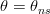$\theta=\theta_{ns}$

where:$\theta=\theta_{s}$ is the water content in the saturated soil (m3/m3)$\theta=\theta_{ns}$ is the water content in the non saturated soil (m3/m3)

In order to calculate field capacity by the equation (1.7) the evaluation of the suction head is needed :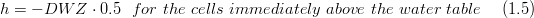$h=-DWZ\cdot 0.5\,\,\,\,for\,\, the\,\, cells\,\, immediately\,\, above\,\, the\,\, water\,\, table \,\,\,\,\,\,\,\, (1.5)$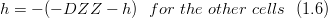$h=-(-DZZ-h)\,\,\,\,for\,\, the\,\, other\,\, cells\,\,\,\, (1.6)$

As shown in the picture the suction head is calculated in order to maintain the same total head (H = z + p + h) in the cells in agreement with the field capacity definition.

### Water retention

The model use for characterizing the shape of water retention curves is the van Genuchten model: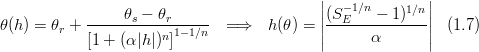$\theta(h) = \theta_r + \frac{\theta_s - \theta_r}{\left[ 1+(\alpha |h|)^n \right]^{1-1/n}}\,\,\,\,\Longrightarrow\,\,\,\,h(\theta)=\left |\frac{(S_{E}^{-1/n}-1)^{1/n}}{\alpha} \right |\,\,\,\,(1.7)$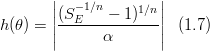$h(\theta)=\left |\frac{(S_{E}^{-1/n}-1)^{1/n}}{\alpha} \right |\,\,\,\,(1.7)$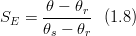$S_{E}=\frac{\theta-\theta_{r}}{\theta_{s}-\theta_{r}}\,\,\,\,(1.8)$

where: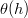$\theta(h)$ is the the water retention curve (m 3/m 3)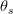$\theta_s$ is the saturated water content (m 3/m 3)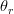$\theta_r$ is the residual water content (m 3/m 3) h is the suction head (m)$\alpha$ is related to the inverse of the air entry (m -1) n is a measure of the pore-size distribution n>1 (dimensionless)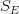$S_{E}$ is the effective saturation (dimensionless)

### Saturated and Unsaturated Conductivity

The saturated conductivity is given depending on the type of soil; instead the unsaturated conductivity is obtained from the suction head by the Maulem model: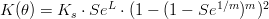$K(\theta)=K_{s}\cdot Se^{L}\cdot (1-(1-Se^{1/m})^{m})^{2}$ (1.9)

where: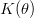$K(\theta)$ is the unsaturated conductivity (m/s)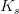$K_{s}$ is the saturated conductivity(m/s) L empirical pore-connectivity (m) m m=1-1/n(dimensionless)

### Evapotranspiration

Some water may be extracted from the soil because of the evaporation and transpiration processes, which become a sink in soil water profile. These two processes are currently named Evapotranspiration.

Potential Evapotranspiration may be modeled using the Penmann Monteith equation. Also, if vegetation exists, a differentiation between Potential Transpiration and Potential Evaporation is done using LAI. These computation are made in Module Basin since this is the module that handles water fluxes in the interface betwen modules.

However, not all of the potential water that can be evaporated or transpired will be in fact removed from the soil. The water that will really leave the soil through these processes is calculated: i) if vegetation exists, effective transpiration is computed in module Vegetation; ii) effective evaporation is computed in the Porous Media module. In Figure below it can be seen that the actual transpiration and evaporation are then used in Porous Media module to compute the new water content. The actual evaporation, which happens only at the soil surface, is calculated based on: i) a pressure head limit or ii) a soil conductivity limit, chosen by the user. It allows the model not to evaporate any surface water, even if it is available, when the soil head gets below the assigned value (i) or limits evaporation velocity to layer unsaturated conductivity (ii).

Remind that Feddes is one option for computing effective transpiration in plants in module Vegetation.

### Iteration Process

Once obtained the water fluxes a balance on the water volume of each cell is apply in order to obtain the new water content$\theta$. The balance applied is the following:

Horizontal Direction X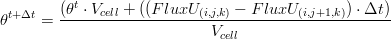$\theta^{t+\Delta t}= \frac {(\theta^{t}\cdot V_{cell}+((FluxU_{(i,j,k)} - FluxU_{(i,j+1,k)})\cdot \Delta t)} { V_{cell}}$

Horizontal Direction Y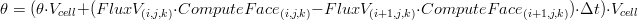$\theta=(\theta\cdot V_{cell}+(FluxV_{(i,j,k)}\cdot ComputeFace_{(i,j,k)}-FluxV_{(i+1,j,k)}\cdot ComputeFace_{(i+1,j,k)})\cdot \Delta t)\cdot V_{cell}$

Vertical Direction W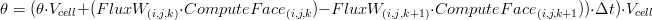$\theta=(\theta\cdot V_{cell}+(FluxW_{(i,j,k)}\cdot ComputeFace_{(i,j,k})-FluxW_{(i,j,k+1)}\cdot ComputeFace_{(i,j,k+1}))\cdot \Delta t)\cdot V_{cell}$

Transpiration Flux$\theta=(\theta\cdot V_{cell}-(TranspFlux_{(i,j,k)}\Delta t)\cdot V_{cell}$

Evaporation Flux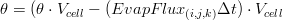$\theta=(\theta\cdot V_{cell}-(EvapFlux_{(i,j,k)}\Delta t)\cdot V_{cell}$

Infiltration Flux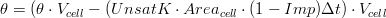$\theta=(\theta\cdot V_{cell}-(UnsatK\cdot Area_{cell}\cdot(1-Imp) \Delta t)\cdot V_{cell}$

where:$\theta$ is the water content (m 3/m 3)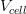$V_{cell}$ is the cell volume (m 3)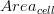$Area_{cell}$ is the cell area (m 2) ComputeFace is the computed face (dimensionless)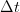$\Delta t$ is the time step (s) FluxX is the flux in X direction (m 3/s) FluxV is the flux in Y direction (m 3/s) FluxW is the flux in W direction (m 3/s) TranspFlux is the transpiration flux (m 3/s) EvapFlux is the evaporation flux (m 3/s) UnsatK is unsaturated conductivity (m /s) Imp is the percentage of impermeable soil of the cell (dimensionless)

The value of$\theta$ so obtained is compared with one used in the volumes calculation and the iterative process stop when:

$'})-(\theta^{new$ (1.10)

where: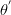$\theta^{'}$ is the water content of the previous iteration (m 3/m 3)

If the equation (1.10) is not satisfy the temporal step is divided in half and the new value of$\theta$ is used for solving the equation (1.7) for restarting the calculation process. The iteration process is stoped when the tolerance desired is reached.

## Boundary Conditions

In PorousMedia there is the option to define the boundary condition in different components. It can be imposed an aquifer level at the soil lateral "walls" and/or free flux in the bottom.

The level imposed in lateral walls is used to compute lateral flows using the same equation as for soil (Buckingham-Darcy). In the outside (boundary) it is assumed that field capacity occurs above aquifer and no hidrostatic pressure in saturated area (the total head is the same in all column).

For the bottom boundary condition is assumed "free-flow" or "null gradient" where water content is the same in both bottom and outside. It is assumed no hidrostatic pressure in the bottom what is reasonable since water is moving trough the bottom.

### Computation

#### Lateral Boundary

The boundary fluxes are computed after the flow computation iteration. Boundary flows are computed in cells that are saturated and higher than boundary level or cells unsaturated lower than boundary level.

Lateral Boundary flux is computed with Buckingham-Darcy equation in all faces that are boundary using saturated conductivity (saturated front movement).

The lateral boundary level can be imposed as a constant value everywhere or defined by piezometers where level data can be interpolated to boundary in space and time. In case of piezometers the user provides the location coordinates and the model interpolates (using triangulation or IWD) the level data (single value or timeserie) to the boundary cells.

#### Bottom Boundary

Bottom Boundary flux is computed using bottom conductivity derived from Buckingham-Darcy equation (Head gradient is one) and flux is:

ConductivityBottom * Area


### Keywords

#### Lateral Boundary

The keyword in PorousMedia_X.dat that connects the lateral open boundary is:

IMPOSE_BOUNDARY_VALUE     : 1


The keyword that defines the lateral boundary level (constant everywhere) is:

BOUNDARY_VALUE            : 100.


The lateral open boundary computation can be limited to specific areas defining the maximum altimetry that the boundary will be open. This is specifically useful when one wants to open the groundwater water at the end of the watershed where in fact the flux can go trough the boundaries delimitation.

MAX_DTM_FOR_BOUNDARY      : 1000.


Using a value of the latter keyword higher than the maximum altimetry found in the watershed will make the boundary open in all watershed.

The blocks that allows to define piezometers (if not defined, the value used is BOUNDARY_VALUE everywhere.

<begin_boundary>
INTERPOLATION_METHOD      : 1   !1 triangulation; 2- IWD
WRITE_TRIANGLES           : 1
TRIANGLES_FILE            : ..\General Data\Boundary Conditions\Triangles.xy
<<begin_piezometer>>
NAME                      : Piezometer1
COORD_X                   : 1.2250
COORD_Y                   : 43.8745
VALUE_TYPE                : CONSTANT
DEFAULTVALUE              : 100
!VALUE_TYPE               : TIMESERIE
!FILENAME                 : ..\General Data\Boundary Conditions\Piezometer1.dat
!DATA_COLUMN              : 2
<<end_piezometer>>
...
...
<end_boundary>


For triangulation is needed at least 3 piezometers as minimum.

#### Bottom Boundary

The keyword in PorousMedia_X.dat that connects the bottom open boundary is:

IMPOSE_BOUNDARY_BOTTOM    : 1


## Discharges

In PorousMedia the discharges may be positive or negative (PorousMedia uses discharge flow) and are dealt with ModuleDischarges. It was only programmed in PorousMedia the using of the defined discharge.

To have a discharge in PorousMedia the user defines a discharge in any K_CELL positive and different from zero in the discharge definition (Discharges_X.dat.

### Computation

The discharges are initialized in Construct phase reading its locations and checking if inside boundaries.

In Modification phase, the several discharges flows are accounted and water content updated. The computation is inside the water content iteration process. And integration variable integrates this flow for PorousMediaProperties.

### Keywords

For discharges to be read in Discharges_X.dat the following keyword needs to exist in the PorousMedia_X.dat

DISCHARGES : 1


Without these keyword no matter what is inside Discharges_X.dat it will not be read!

## Other Features

### How to Generate Info needed in Porous Media

#### SoilMap

Model needs to know soil ID in each cell and layer to pick hydraulic properties from that type of soil. In Pedology soil includes more than one horizon, each with different soil properties. Here Soil is used has a unit of soil hidraulic properties, i.e., to define a soil with three horizons one has to create three SoilID (see below). This also means that if a watershed has at least one soil with three horizons one has to create three soil maps. Each soil map will be infact the map of each horizon of the soils. The grid cells with only one horizon will have the same SoilID in all maps, the grid cells with three horizons will have a different SoilID in each map.

Options:

• Constant value
• Soil Grid. One possible option is to associate with soil shape file. In this case can use MOHID GIS going to menu [Tools]->[Shape to Grid Data] and provide: i) the grid (model grid), ii) the soil shape file and iii) the corespondence between soil codes and soil ID defined in data file.

Soil ID must be defined in Module FillMatrix standards for each soil horizon defined (grid example):

<beginhorizon>
KLB                       : 1
KUB                       : 10


<beginproperty>
NAME                      : SoilID
DEFAULTVALUE              : 1
INITIALIZATION_METHOD     : ASCII_FILE
REMAIN_CONSTANT           : 1
FILENAME                  : ..\..\GeneralData\PorousMedia\SoilID200m.dat
<endproperty>

<beginproperty>
<endproperty>
..
<endhorizon>


Remarks

All the soil ID's appearing in the soil grid(s) must be defined in the PorousMedia data file in terms of hydraulic properties:

<beginsoiltype>
ID                        : 1
THETA_S                   : 0.3859      !is the saturated water content (m 3/m 3) - equation 1.7 (theta s)
THETA_R                   : 0.0476      !is the residual water content (m 3/m 3) - equation 1.7  (theta r)
N_FIT                     : 1.39        !is a measure of the pore-size distribution n>1 (dimensionless)  - equation 1.7 (n)
SAT_K                     : 3.5556e-6   !is the saturated conductivity(m/s) - equation 1.8 (Ks)
ALPHA                     : 2.75        !is related to the inverse of the air entry (m -1) - equation 1.7 (alpha)
L_FIT                     : 0.50        !empirical pore-connectivity (m) - equation 1.8 (l)
<endsoiltype>

<beginsoiltype>
ID                        : 2
...
<endsoiltype>
...


#### Soil Bottom

The soil depth must be known by the model. This is computed by the model from terrain altitude (topography) and soil bottom altitude. As so, a soil bottom grid is needed.

Options:

• Grid File.

Soil depth (and soil bottom altitude, the effective grid needed) can be defined with a constant depth or estimated from slope HOW TO SoilBottom LINK. When the soil depth is estimated as a function of slope, soil depth will be smaller in ares with higher slope. In this areas only the surface layers of the soil will be considered (see Porous Media Geometry).

Define the grid just generated, in the porous media data file with:

BOTTOM_FILE  : ..\..\GeneralData\PorousMedia\BottomLevel.dat


#### Water Level

Options:

• Grid File.

The water table altitude represents the initial altitude of the water table. It is recommended to do a spin-up run to estabilize water level and then do a continuous simulation starting with the final water table achieved. Use the following blocks with Module FillMatrix standards:

<beginwaterlevel>
NAME                      : waterlevel
INITIALIZATION_METHOD     : ASCII_FILE
DEFAULTVALUE              : 0
REMAIN_CONSTANT           : 0
FILENAME                  : ..\..\GeneralData\PorousMedia\WaterLevel0.50.dat
<endwaterlevel>


#### Impermeability

Impermeability values (0 - completely permeable, 1 - impermeable) must be provided.

Options:

• Constant Value.
• Grid File. One possible option is to associate with land use shape file. In this case can use MOHID GIS going to menu [Tools]->[Shape to Grid Data] and provide: i) the grid (model grid), ii) the land use shape file and iii) the corespondence between land use codes and Impermeability values.

Use the following blocks with Module FillMatrix standards:

<beginimpermeablefraction>
NAME                      : impermeablefraction
INITIALIZATION_METHOD     : ASCII_FILE
DEFAULTVALUE              : 0
REMAIN_CONSTANT           : 1
FILENAME                  : ..\..\GeneralData\PorousMedia\AreaImpermeavel.dat
<endimpermeablefraction>


## Outputs

### Timeseries

Theta - is water content of selected cell (vol water/ vol soil)

relative_water_content - content in selected cell. between zero and one (zero is residual water content and one is saturated water content)

VelW_[m/s] - vertical velocity in the bottom face of the selected cell

VelW_Corr_[m/s] - vertical velocity in the bottom face of the selected cell that may be corrected if oversaturation occurs. if no correction occurs is the same as previous.

InF_Vel_[m/s] - infiltration velocity (in the soil surface)

Head_[m] - Suction in selected cell

Conductivity_[m/s] - Conductivity in selected cell

level_water_table_[m] - water table altitude

water_table_depth_[m] - water table depth (from soil surface)

Hydro_Pressure_[m] - hydrostatic pressure in selected cell

Final_Head_[m] - Soil water charge in selected cell

[Check Mohid Land Heights and Levels to understand some of the outputs]

GW_flow_to_river_total_[m3/s] - Ground water flow to river if it is a river point

Surface_Evaporation_Flux_[m3/s] - evaporation flux (in the soil surface)

Transpiration_Flux_[m3/s] - transpiration flux in selected cell